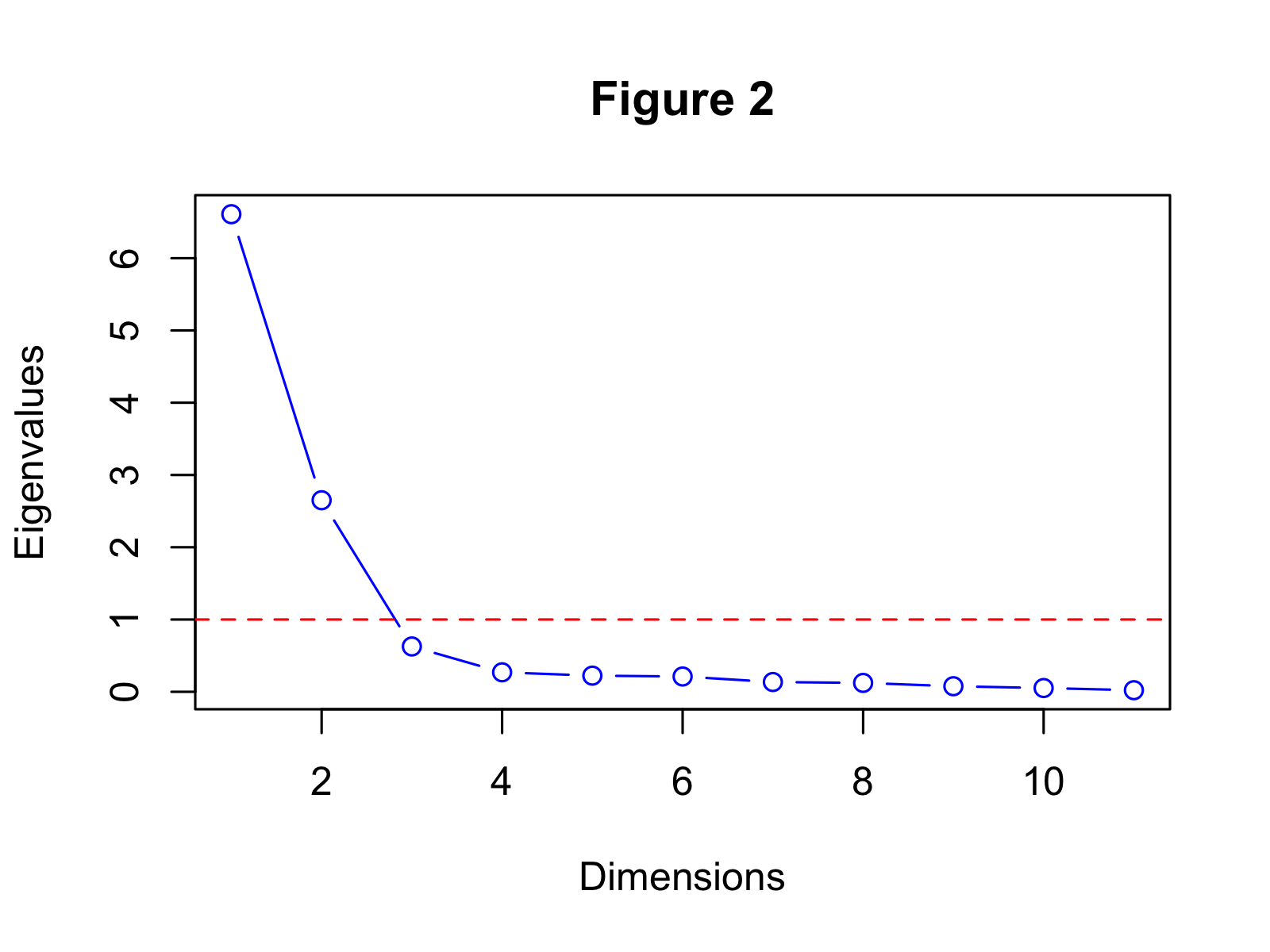# Scree Plot for PCA Explained

When we perform a Principal Component Analysis (PCA), the main idea is to capture the most of the variance of our data using a lower-dimensional space. To accomplish this, we use newly defined components, which are lower in numbers than the original variables.

But how much lower? One way of deciding on this is to use a scree plot. In this tutorial, you’ll learn how to interpret a scree plot in a PCA.

This page was created in collaboration with Paula Villasante Soriano and Cansu Kebabci. Please have a look at Paula’s and Cansu’s author pages to get further information about their academic backgrounds and the other articles they have written for Statistics Globe.

Let’s dive right in.

## What is Scree Plot?

Scree plot is a graphic that shows the explained variance per newly defined component (principal component). The measure of the plot can be the percentage or the absolute value of the explained variance (eigenvalues). For the demonstration, we used the built-in mtcars data and the measure of explained variance percentage. See Figure 1 below.In Figure 1, the x-axis shows the principal components (dimensions), which are 10 in this case. The y-axis shows the percentage of the explained variance per principal component.

It is seen that a large proportion of variance (60.1%) is explained by the first principal component, whereas the relatively smaller proportions of variance (24.1%, 5.7%, ..) are explained by the rest of the components. It’s common in practice that the first principal component explains the major amount of variance.

But how does this visual help to decide on the number of components to keep? Let’s see it in the next section!

## Number of Components to Keep

In order to decide how many components to keep after creating our scree plot, there’s two different methods we can use:

### The Elbow Method

A method of interpreting a scree plot is to use the elbow rule. This method is about looking for the “elbow” shape on the curve and retaining all components before the point, where the curve flattens out.

In Figure 1, the elbow appears to occur at the second principal component. This means that the first two components should be kept for the analysis.

### Kaiser’s Rule

Kaiser’s rule is a commonly used method to select the number of components in a PCA. It’s based on keeping the components with eigenvalues greater than 1.

If we create the scree plot by showing the eigenvalues this time, the graph will look like in Figure 2 :We can see that the first two principal components have eigenvalues greater than 1. Thus, this method also leads us to keep two components.

As shown, the elbow method and Kaiser’s rule help us to decide on the number of components to keep. However, those are rather subjective methods. For instance, in the presence of multiple “elbows”, it would be harder to decide on the optimal number of components when using the elbow method. For this reason, you might be interested in looking at other ways to choose the optimal number of principal components.

## Video, Further Resources & Summary

Do you need more explanations on how to understand your scree plot? Then you should have a look at the following YouTube video of the Statistics Globe YouTube channel.

There are other tutorials on Statistics Globe you can take a look at:

This post has shown how to interpret scree plots in PCA. In case you have further questions, don’t hesitate to leave a comment.

Subscribe to the Statistics Globe Newsletter